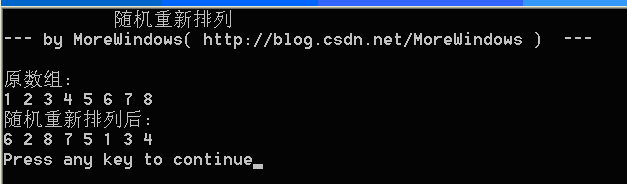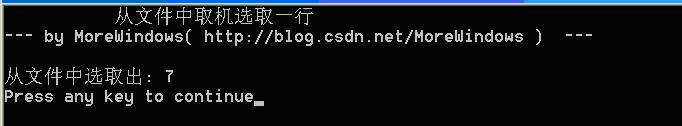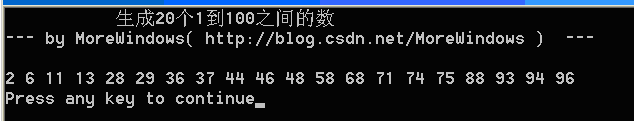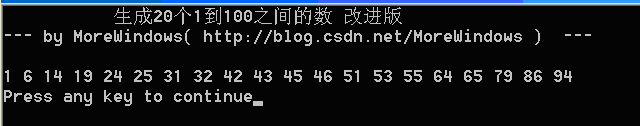## 一．随机重新排列

if (rand() % 2 == 0)

swap(a, a)

1．先调整前二个元素即{1, 23}先生成{123}{213}

2．然后对{123}，第三个元素以1/3的概率与第一，第二，第三个元素进行交换就可以等概率的得到{321}{132}{123}

3．同理对{213}，可以等概率的得到{312}{231}{213}

```//随机重新排列
//参照STL中的random_shuffle
#include <stdio.h>
#include <stdlib.h>
#include <time.h>
inline void Swap(int *a, int *b)
{
int c = *a;
*a = *b;
*b = c;
}
//随机重新排列函数
void Random_shuffle(int a[], int n)
{
srand(time(NULL));
for (int i = 1; i < n; i++)
Swap(&a[i], &a[rand() % (i + 1)]);
}
void PrintfIntArray(int a[], int n)
{
for (int i = 0; i < n; i++)
printf("%d ", a[i]);
putchar('\n');
}
int main()
{
printf("           随机重新排列 \n");
printf("--- by MoreWindows( http://blog.csdn.net/MoreWindows )  ---\n\n");

const int MAXN = 8;
int a[MAXN] = {1, 2, 3, 4, 5, 6, 7, 8};

printf("原数组:\n");
PrintfIntArray(a, MAXN);

Random_shuffle(a, MAXN);

printf("随机重新排列后:\n");
PrintfIntArray(a, MAXN);
return 0;
}```## 二．从文件中随机取一行数据

```//从文件中取机选取一行
#include <stdio.h>
#include <stdlib.h>
#include <time.h>
int main()
{
printf("           从文件中取机选取一行 \n");
printf("--- by MoreWindows( http://blog.csdn.net/MoreWindows )  ---\n\n");

int i, num, nChooseNum;
const char strFileName[] = "in.txt";

freopen(strFileName, "r", stdin);
srand(time(NULL));
i = 1;
while (scanf("%d", &num) != EOF)
{
if (rand() % i == 0)
nChooseNum = num;
i++;
}
printf("从文件中选取出: %d\n", nChooseNum);
return 0;
}```## 三．生成N个随机数

```//生成n个指定范围的随机数
#include <stdio.h>
#include <time.h>
#include <set>
//生成n个[s, e)范围的数
void GetRandNumberInRange(int *a, int n, int s, int e)
{
int   i, j;
std::set<int>  m;
std::set<int>::iterator setpos;

srand(time(NULL));
while (m.size() < n)
{
j = rand() % (e - s) + s;
m.insert(j);
}

i = 0;
for (setpos = m.begin(); setpos != m.end(); setpos++)
a[i++] = *setpos;
}
void PrintfIntArray(int a[], int n)
{
for (int i = 0; i < n; i++)
printf("%d ", a[i]);
putchar('\n');
}
int main()
{
const int NMAX = 20;
const int NUMSTART = 1;
const int NUMEND = 100;

printf("           生成%d个%d到%d之间的数 \n", NMAX, NUMSTART, NUMEND);
printf("--- by MoreWindows( http://blog.csdn.net/MoreWindows )  ---\n\n");

int    a[NMAX];

GetRandNumberInRange(a, NMAX, NUMSTART, NUMEND + 1);
PrintfIntArray(a, NMAX);
return 0;
}``````//生成n个指定范围的随机数
#include <set>
#include <cstdio>
#include <cstdlib>
#include <ctime>
//在[s, e)区间上随机取n个数并存放到a[]中
void GetRandomNum(int *a, int n, int s, int e)
{
std::set<int> set_a;
srand(time(NULL));
for (int i = e - n; i < e; i++)
{
int num = (rand() % i) + s;
if (set_a.find(num) == set_a.end())
set_a.insert(num);
else
set_a.insert(i);
}
i = 0;
std::set<int>::iterator pos;
for (pos = set_a.begin(); pos != set_a.end(); pos++)
a[i++] = *pos;
}
void PrintfIntArray(int a[], int n)
{
for (int i = 0; i < n; i++)
printf("%d ", a[i]);
putchar('\n');
}
int main()
{
const int NMAX = 20;
const int NUMSTART = 1;
const int NUMEND = 100;

printf("           生成%d个%d到%d之间的数 改进版\n", NMAX, NUMSTART, NUMEND);
printf("--- by MoreWindows( http://blog.csdn.net/MoreWindows )  ---\n\n");
int a[NMAX];

GetRandomNum(a, NMAX, NUMSTART, NUMEND + 1);
PrintfIntArray(a, NMAX);
return 0;
}```